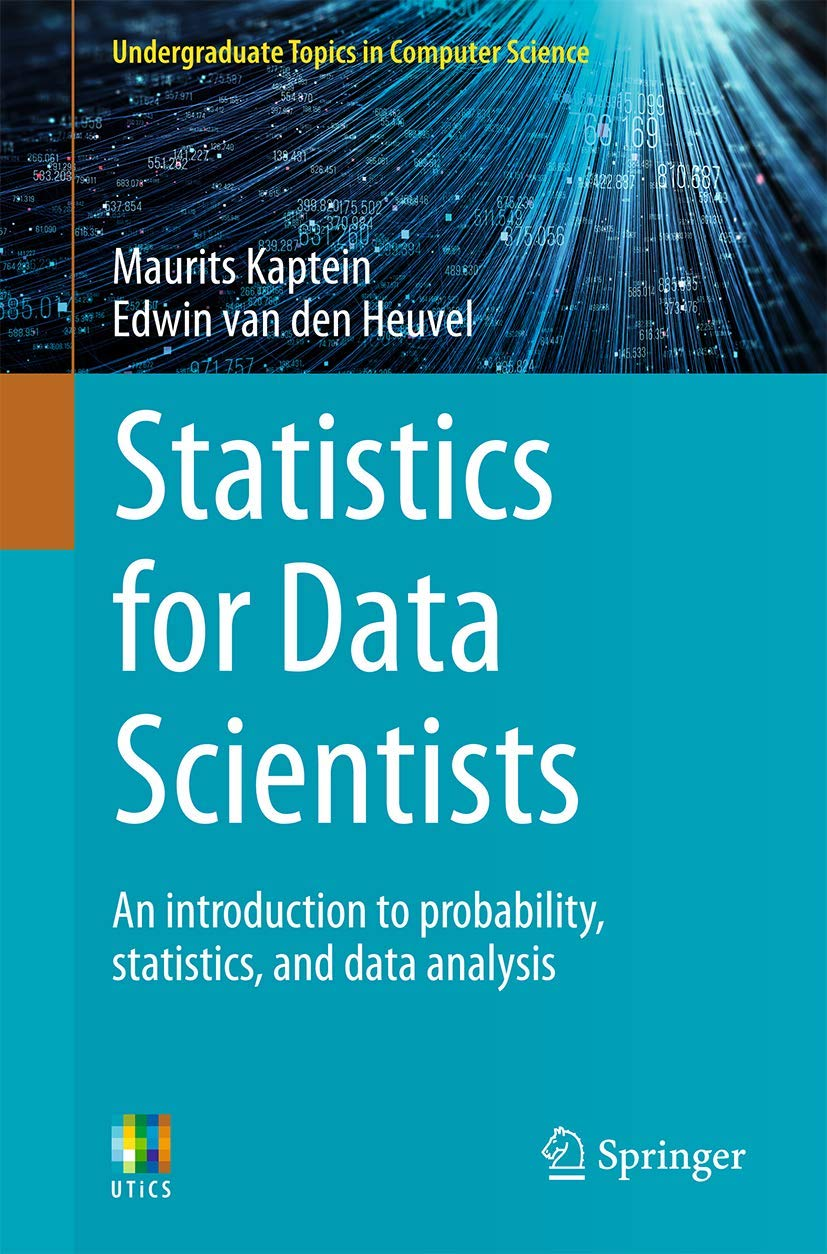# Statistics for Data Scientists: An Introduction to Probability, Statistics, and Data Analysis# Statistics for Data Scientists: An Introduction to Probability, Statistics, and Data Analysis

• Length: 345 pages
• Edition: 1
• Language: English
• Publisher: Springer
• Publication Date: 2021-02-11

This book provides an undergraduate introduction to analysing data for data science, computer science, and quantitative social science students. It uniquely combines a hands-on approach to data analysis – supported by numerous real data examples and reusable [R] code – with a rigorous treatment of probability and statistical principles.

Where contemporary undergraduate textbooks in probability theory or statistics often miss applications and an introductory treatment of modern methods (bootstrapping, Bayes, etc.), and where applied data analysis books often miss a rigorous theoretical treatment, this book provides an accessible but thorough introduction into data analysis, using statistical methods combining the two viewpoints. The book further focuses on methods for dealing with large data-sets and streaming-data and hence provides a single-course introduction of statistical methods for data science.

#### Tận hưởng giấc ngủ ngon với Nghe truyện thiền Khúc sông thanh bình